模型保存与加载_仅保存和加载模型参数和保存和加载整个模型的区别 - CSDN

• tensorflow提供了Saver类用于模型保存与导入。该类定义在tensorflow/python/training/saver.py.中。 Saver类的默认初始化函数如下： __init__( var_list=None, reshape=False, sharded=False, max_to_keep...
tensorflow版本为1.4.1

tensorflow提供了Saver类用于模型的保存与导入。该类定义在tensorflow/python/training/saver.py.中。

Saver类的默认初始化函数如下：

__init__(
var_list=None,
reshape=False,
sharded=False,
max_to_keep=5,
keep_checkpoint_every_n_hours=10000.0,
name=None,
restore_sequentially=False,
saver_def=None,
builder=None,
defer_build=False,
allow_empty=False,
write_version=tf.train.SaverDef.V2,
save_relative_paths=False,
filename=None
)

由于该初始化函数均有缺省值，因此我们常用的创建一个Saver对象的操作为tf.train.Saver()

下面解释一下常用的参数：

var_list: Variable/SaveableObject的列表，或者是一个字典(mapping names to SaveableObjects)。默认为None，即保存所有可保存的对象。
reshape: 当为True时，表示从一个checkpoint中恢复参数时允许参数shape发生变化。当我们reshape了一个变量又希望加载旧模型时，该操作就很有用。
max_to_keep:为了避免填满整个磁盘，Saver可以自动的管理Checkpoints文件。该参数用于指定保存最近的N个Checkpoints文件，默认为5.
keep_checkpoint_every_n_hours: 为了避免填满整个磁盘，Saver可以自动的管理Checkpoints文件。该参数用于指定保留Checkpoints文件的时间，默认为10000小时
保存模型需要用到save函数：

save(
sess,
save_path,
global_step=None,
latest_filename=None,
meta_graph_suffix='meta',
write_meta_graph=True,
write_state=True
)

下面介绍其中的参数：

sess: 保存模型要求必须有一个加载了计算图的会话，而且所有变量必须已被初始化。
save_path: 模型保存路径及保存名称。
global_step: 如果提供的话，这个数字会添加到save_path后面，用于区分不同训练阶段的结果。
加载模型的函数为：

restore(
sess,
save_path
)

sess: 加载模型要求必须有一个加载了计算图的会话，但是不要求变量初始化。
save_path: 模型保存路径
下面给一个模型保存的例子，例子还是使用 “单层感知机实现mnist数字分类”

# -*- coding: utf-8 -*-
import tensorflow as tf
import os

# don't show INFO
os.environ['TF_CPP_MIN_LOG_LEVEL'] = '1'
# single layer perceptron: y = wx + b
# input
x = tf.placeholder(tf.float32, [None, 784])
# weights
W = tf.Variable(tf.random_normal([784,10], stddev=0.1))
# bias
b = tf.Variable(tf.zeros())
# softmax
y = tf.nn.softmax(tf.matmul(x,W) + b)
# output
y_ = tf.placeholder(tf.float32, [None, 10])
# cross_entropy loss
cross_entropy = tf.reduce_mean(-tf.reduce_sum(y_ * tf.log(y), reduction_indices=))
# optimization with gradient descend, the learning rate is set as 0.02
# initalize all variables
init = tf.global_variables_initializer()
# start a new session
sess = tf.Session()
sess.run(init)
m_saver = tf.train.Saver()

# 2000 iterations
for i in range(2000):
batch_xs, batch_ys = mnist.train.next_batch(100)
sess.run(train_step, feed_dict={x: batch_xs, y_: batch_ys})
if i % 100 == 0:
m_saver.save(sess, './model/mnist_slp', global_step=i)

# computer the accuracy
correct_prediction = tf.equal(tf.argmax(y,1), tf.argmax(y_,1))
accuracy = tf.reduce_mean(tf.cast(correct_prediction, tf.float32))
print(sess.run(accuracy, feed_dict={x: mnist.test.images, y_: mnist.test.labels}))

# close the session
sess.close()

最后可以在model文件下看到保存的文件：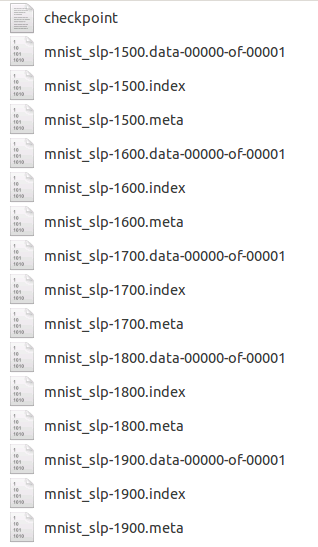说明：

尽管每100次保存一次模型，但实际上只会保存最近的5次。
每次保存都会产生3个文件，其中mnist_slp-1900.data-00000-of-00001存放的是模型参数，mnist_slp-1900.meta中存放的是计算图。
当我们加载模型时，如下：

# -*- coding: utf-8 -*-
import tensorflow as tf
import os

# don't show INFO
os.environ['TF_CPP_MIN_LOG_LEVEL'] = '1'

# single layer perceptron: y = wx + b
# input
x = tf.placeholder(tf.float32, [None, 784])

# weights
W = tf.Variable(tf.random_normal([784,10], stddev=0.1))

# bias
b = tf.Variable(tf.zeros())

# softmax
y = tf.nn.softmax(tf.matmul(x,W) + b)

# output
y_ = tf.placeholder(tf.float32, [None, 10])

# cross_entropy loss
cross_entropy = tf.reduce_mean(-tf.reduce_sum(y_ * tf.log(y), reduction_indices=))

# optimization with gradient descend, the learning rate is set as 0.02

# start a new session
sess = tf.Session()

m_saver = tf.train.Saver()

m_saver.restore(sess, './model/mnist_slp-1900')

# computer the accuracy
correct_prediction = tf.equal(tf.argmax(y,1), tf.argmax(y_,1))
accuracy = tf.reduce_mean(tf.cast(correct_prediction, tf.float32))
print(sess.run(accuracy, feed_dict={x: mnist.test.images, y_: mnist.test.labels}))

# close the session
sess.close()
展开全文tensorflow
• 模型保存与加载的两种方式
模型保存与加载的两种方式
保存模型的两种方式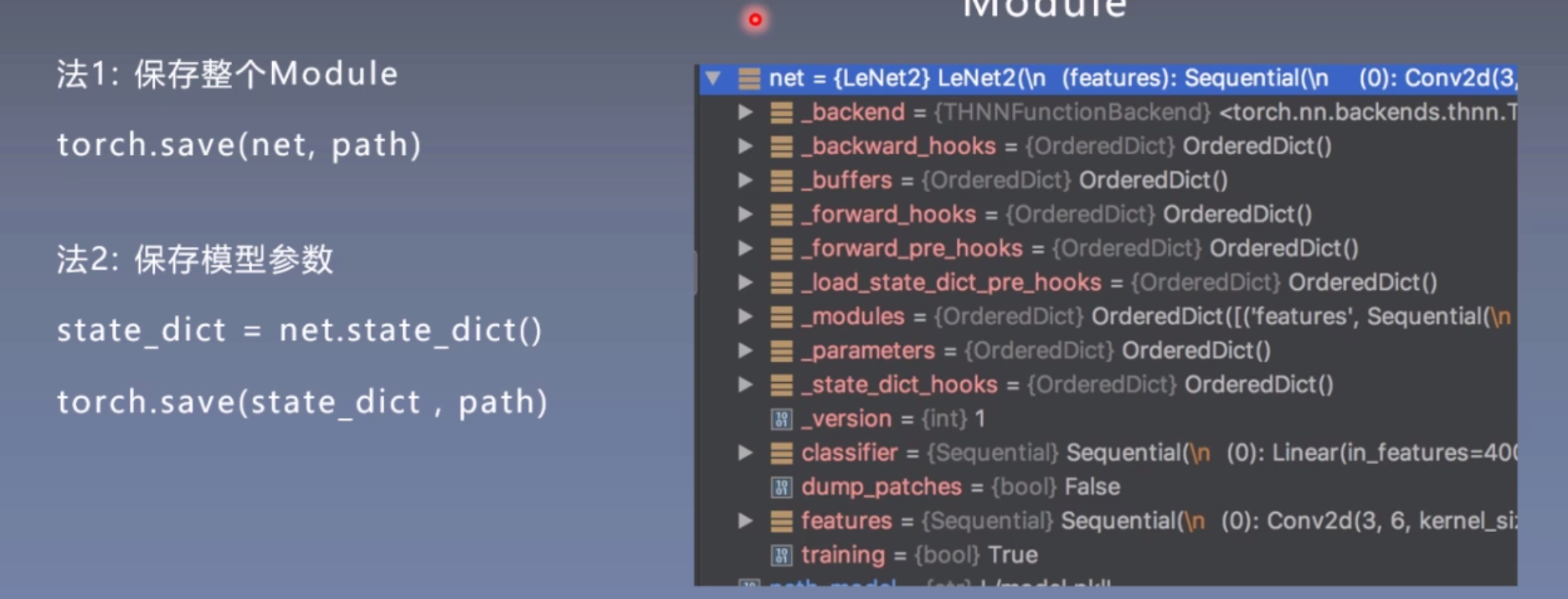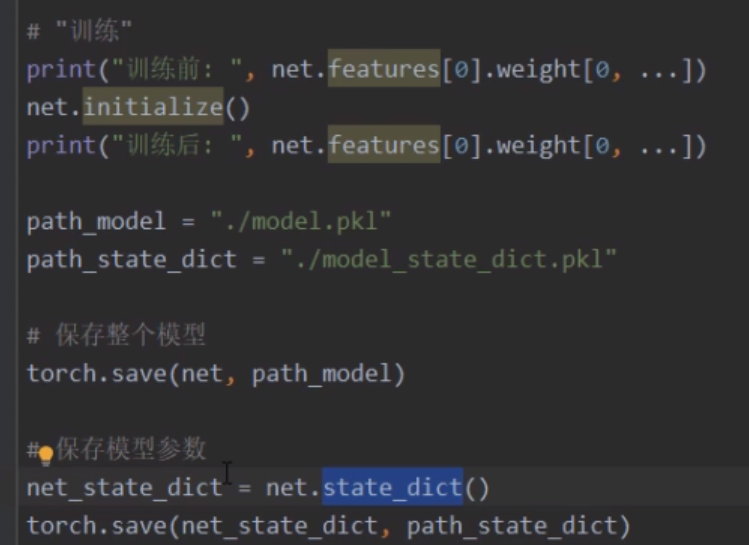加载
方式一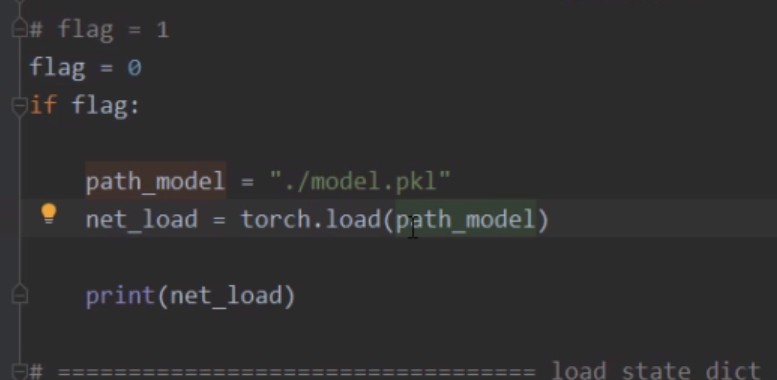方式二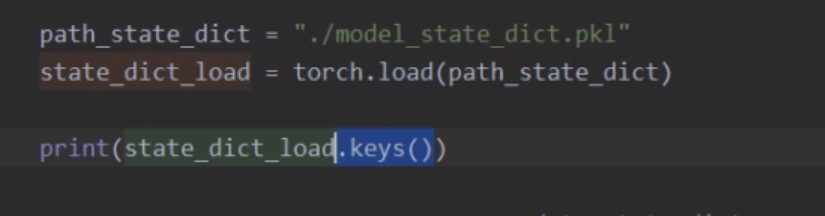展开全文• 刚接触深度学习，Tensorflow模型保存加载尚不清楚，根据教程的翻译做一记录，不当之处敬请指正。原文地址：http://cv-tricks.com/tensorflow-tutorial/save-restore-tensorflow-models-quick-complete-tutorial/...
刚接触深度学习，Tensorflow模型的保存和加载尚不清楚，根据教程的翻译做一记录，不当之处敬请指正。原文地址：http://cv-tricks.com/tensorflow-tutorial/save-restore-tensorflow-models-quick-complete-tutorial/在本教程中，将作出如下讲解：    I.   Tensorflow模型是什么样的？    II.  如何保存一个训练好的Tensoflow模型？    III. 如何加载一个先前保存的Tensorflow模型？    IV. 如何对pretrained模型进行fine-tuning和修改？本教程假设你已经对神经网络的训练有了一定的基础。1.Tensorflow的模型到底是什么样的？：在训练好一个神经网络模型之后，我们通常希望将它保存下来，方便以后的使用。那么，什么是Tensorflow模型呢？Tensorflow模型主要包含网络的设计（图）和训练好的各参数的值等。所以，Tensorflow模型有两个主要的文件：a) Meta graph:这是一个协议缓冲区(protocol buffer)，它完整地保存了Tensorflow图；即所有的变量、操作、集合等。此文件以 .meta 为拓展名。b) Checkpoint 文件：这是一个二进制文件，包含weights、biases、gradients 和其他所有变量的值。此文件以 .ckpt 为扩展名. 但是，从Tensorflow 0.11版本之后做出了一些改变。现在，不再是单一的 .ckpt 文件，而是一下两个文件：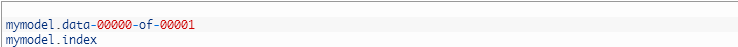.data文件包含了我们的训练变量，稍后再说。另外，Tensorflow还有一个名为 checkpoint 的文件，仅用于保存最新checkpoint文件保存的记录。总之，版本0.10以上的Tensorflow模型如下所示：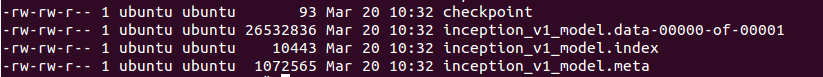注意，Tensorflow 0.11版本之前的模型只有三个文件：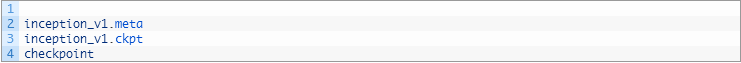现在，我们知道了Tensorflow模型是什么样的，下面我们学习如何保存一个模型。2. 保存一个Tensorflow模型：比方说你正在训练一个卷积神经网络用于图像分类，你会关注于loss值和accuracy. 一旦你看到网络converged， 你就可以手工停止训练或设置固定的训练迭代次数。训练完成之后，我们想把所有变量值和网络图保存到文件中方便以后使用。所以，为了保存Tensorflow中的图和所有参数的值，我们创建一个tf.train.Saver()类的实例。saver = tf.train.Saver()别忘了Tensorflow变量仅存在于session内，所以你必须在session内进行保存，可通过调用创建的saver对象的sava方法实现。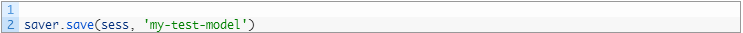其中，sess是session对象，‘my-test-model’是你对自己模型的命名。让我们看一个完整的例子：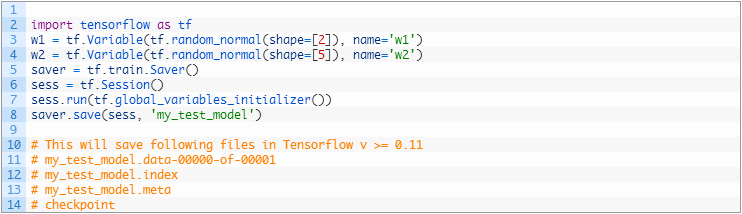如果我们想在迭代1000次后保存模型，我们需在对应的步数之后调用 sava 方法：saver.save(sess, 'my_test_model',global_step=1000)这只是在模型名字后面附加上‘-1000’，同时将创建下列文件：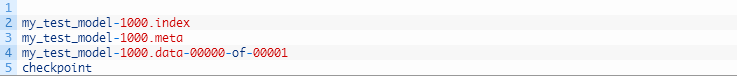比方说，在训练过程中，我们想每迭代1000次就保存模型一次。在第一次保存模型时会创建 .meta 文件(第1000次迭代),并且我们无需每次都重复创建（也就是无需在第2000、3000...或其他迭代时保存.meta文件）。因为图结构不变，我们仅需保存迭代后的参数值。因此，当我们不想写入meat-graph时，使用：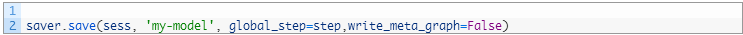如果你想保存最近的4个模型并且每训练两个小时保存一次，可以使用 max_to_keep 和 keep_checkpoint_every_n_hours,如下所示：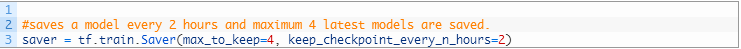注意，如果我们没有在tf.train.Saver()中指定任何参数，它会保存所有变量。如果我们不想保存全部变量而只是想保存一部分的话，我们可以指定想保存的variables/collections.在创建tf.train.Saver实例时，我们将它传递给我们想要保存的变量的列表或字典。看一个例子：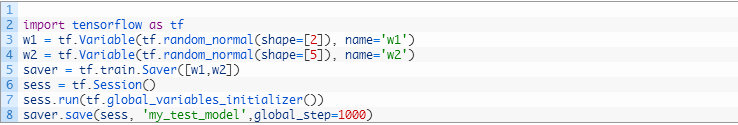这可以用于保存Tensorflow图的特定部分。3.引入一个pretrained模型：如果你想引入其他的预先训练的模型来fine-tuning，需要做两件事：a) 创建网络：你可以通过写python代码手工创建原来模型的网络。或者，通过我们之前创建的 .mate 文件进行网络创建，使用tf.train.import()函数实现，如：saver = tf.train.import_meta_graph('my_test_model-1000.meta')记住，import_meta_graph会将.meta文件中保存的网络加载到当前网络中，这会创建一个graph/network,但我们仍需加载已训练的各参数值。b) 加载参数：我们可以通过调用tf.train.Saver()类的restore方法来加载参数。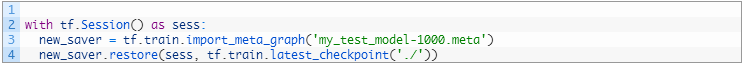这样，像w1、w2这些tensors的值就加载进来了并且可以进行访问：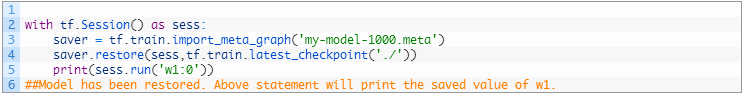现在，你已经知道如何保存和加载Tensorflow模型。下面给出了一个使用练习加载预先训练的模型。。。。
展开全文• 我们可以将训练后的模型保存下来，下次直接导出就行了，节省了时间。 代码： from sklearn.datasets import load_boston from sklearn.model_selection import train_test_split from sklearn.preprocessing ...
我们可以将训练后的模型保存下来，下次直接导出就行了，节省了时间。

代码：

from sklearn.datasets import load_boston
from sklearn.model_selection import train_test_split
from sklearn.preprocessing import StandardScaler
from sklearn.linear_model import Ridge
from sklearn.metrics import mean_squared_error
from sklearn.externals import joblib

def linear3():
"""
岭回归对波士顿房价进行预测
:return:
"""
# 1）获取数据
print("特征数量：\n", boston.data.shape)

# 2）划分数据集
x_train, x_test, y_train, y_test = train_test_split(boston.data, boston.target, random_state=22)

# 3）标准化
transfer = StandardScaler()
x_train = transfer.fit_transform(x_train)
x_test = transfer.transform(x_test)

# 4）预估器
#estimator = Ridge(alpha=0.5, max_iter=10000)
#estimator.fit(x_train, y_train)

# 保存模型
#joblib.dump(estimator, "my_ridge.pkl")
# 加载模型

# 5）得出模型
print("岭回归-权重系数为：\n", estimator.coef_)
print("岭回归-偏置为：\n", estimator.intercept_)

# 6）模型评估
y_predict = estimator.predict(x_test)
print("预测房价：\n", y_predict)
error = mean_squared_error(y_test, y_predict)
print("岭回归-均方误差为：\n", error)

return None

if __name__ == "__main__":
linear3()


展开全文机器学习
• Keras模型保存加载的两种方式方式一: 保存所有状态保存模型和模型图# 保存模型 model.save(file_path) model_name = '{}/{}_{}_{}_v2.h5'.format(params['model_dir'],params['filters'],params['pool_size_1'],...keras
• scikit-learn的基本用法(八)——模型保存与加载sklearn
• ## PyTorch模型保存与加载

万次阅读 多人点赞 2019-05-28 15:04:24Pytorch
• python训练好的模型保存与加载 当我们训练好一个模型model后，如果在其他程序中或者下次想继续使用这个model，我们就需要把这个model保存下来，下次使用时直接导入就好了，不需要重新训练。 方式一： 采用sklearn中...机器学习 python
• 归一化模型保存from sklearn import preprocessing min_max_scaler = preprocessing.MinMaxScaler() X = min_max_scaler.fit_transform(X) from sklearn.externals import joblib joblib.dump(min_max_scaler, '...
• 前言 在使用Pytorch训练模型的时候，经常会有在GPU上保存模型...模型保存与加载 例如我们创建了一个模型： model = MyVggNet() 如果使用多GPU训练,我们需要使用这行代码： model = nn.DataPa...Pytorch
• 本文主要介绍scikit-learn中的模型保存与加载。 Demo 1 import pickle from sklearn.svm import SVC from sklearn import datasets # 定义分类器 svm = SVC() # 加载iris数据集 iris = datasets.load_iris() # ...
• 前面我们已经通过搭建LeNet模型来识别手写数字MNIST，最终一个epoch训练的模型准确率可以达到...整个模型保存与加载 保存模型使用fluid.io.save_inference_model这个API，参数为：保存路径，输入的Variable名，...Paddle
• 模型保存与加载 #多gpu import os os.environ['CUDA_VISIBLE_DEVICES'] = '1,2,3,4' #choose model = TheModelClass(*args, **kwargs) model = torch.nn.DataParallel(model).cuda() #加载与训练模型 file....
• 模型保存与加载       当训练或者计算好一个模型之后，如果别人需要我们提供预测结果，就需要保存模型（主要是保存算法的参数） sklearn模型保存加载API from sklearn....机器学习 sklearnpytorch 模型 保存 加载
• PyTorch使用自定义层预训练模型后，加载模型需要包含当时定义的网络结构
• 本文将会介绍如何利用Keras来实现模型保存、读取以及加载。   本文使用的模型为解决IRIS数据集的多分类问题而设计的深度神经网络（DNN）模型模型的结构示意图如下： 具体的模型参数可以参考文章：Keras入门...Keras HDF5
• ** 一、权重参数的保存与加载 ...权重参数的保存与加载可以针对任何模型，包括自定义的。 但是在加载权重参数时，其模型的结构需要原来的完全一致。 import tensorflow as tf from tensorflow import ker...
• 在TensorFlow中，保存模型与加载模型所用到的是tf.train.Saver()这个类。在加载模型的时候会用到restore()函数我们一般的想法就是，保存模型之后，在另外的文件中重新将模型导入，我可以利用模型中的operation和...restore
• 使用tensorflow过程中，训练结束后我们需要用到模型文件。有时候，我们可能也需要用到别人训练好的模型，并在这个基础上再次训练。这时候我们需要掌握如何操作这些模型数据。看完本文，相信你一定会有收获！Tensorflow
...